# Conduction: One Dimensional - 6 Notes | Study Heat Transfer - Mechanical Engineering

## Mechanical Engineering: Conduction: One Dimensional - 6 Notes | Study Heat Transfer - Mechanical Engineering

The document Conduction: One Dimensional - 6 Notes | Study Heat Transfer - Mechanical Engineering is a part of the Mechanical Engineering Course Heat Transfer.
All you need of Mechanical Engineering at this link: Mechanical Engineering

Conduction: One Dimensional, Heat Transfer

General heat conduction relation in cylindrical coordinate system (fig. 2.12) is derived (briefly) below.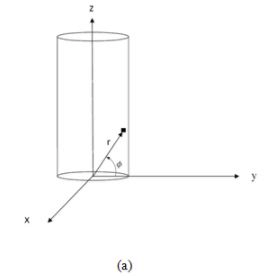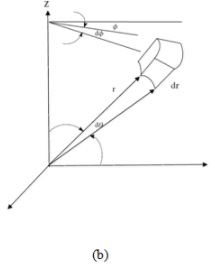Fig.2.12. Cylindrical coordinate system (a) and an element of the cylinder

The energy conservation for the system is written as,

Ӏ + ӀӀ = ӀӀӀ + ӀV     (2.21)
where,
I      : Rate of heat energy conducted in
II    : Rate of heat energy generated within the volume element
III   : Rate of heat energy conducted out
IV   : Rate of energy accumulated (ӀV)
and the above terms are defines as,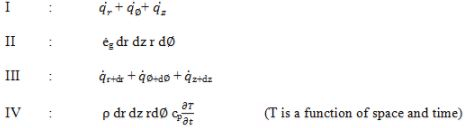Thus,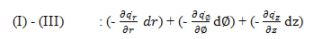###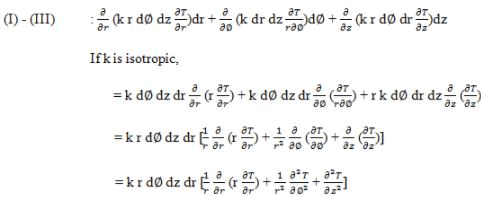On putting the values in equation 2.21,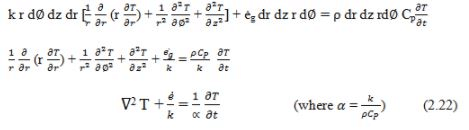Thus the Laplacian operator is,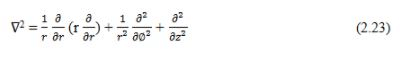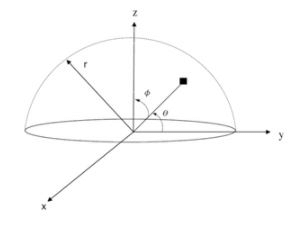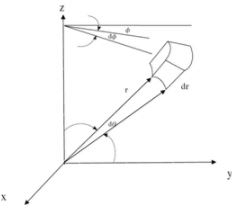(a)                                                                                         (b)

Fig.2.13. Spherical coordinate system (a) and an element of the sphere

In a similar way the general expression for the conduction heat transfer in spherical body with heat source can also be found out as per the previous discussion. The Laplacian operator for the spherical coordinate system (fig.2.13) is given below and the students are encouraged to derive the expression themselves.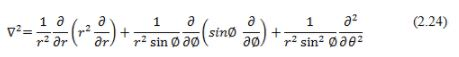The document Conduction: One Dimensional - 6 Notes | Study Heat Transfer - Mechanical Engineering is a part of the Mechanical Engineering Course Heat Transfer.
All you need of Mechanical Engineering at this link: Mechanical EngineeringUse Code STAYHOME200 and get INR 200 additional OFF

## Heat Transfer

58 videos|70 docs|85 tests

Track your progress, build streaks, highlight & save important lessons and more!

,

,

,

,

,

,

,

,

,

,

,

,

,

,

,

,

,

,

,

,

,

;